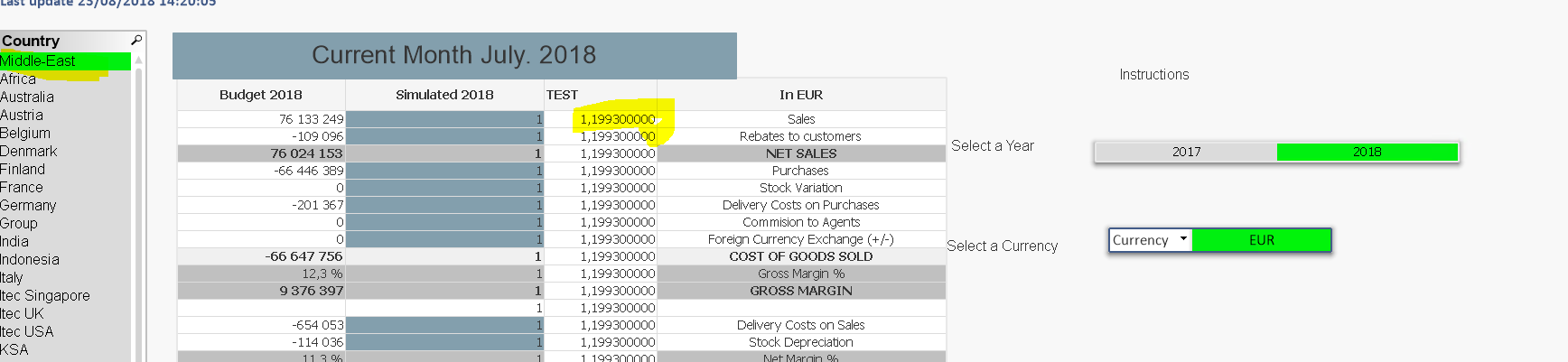# QlikView App Dev

Discussion Board for collaboration related to QlikView App Development.

Announcements
Now Live: Qlik Sense SaaS Simplified Authoring – Analytics Creation for Everyone: READ DETAILS
cancel
Showing results for
Did you mean:Contributor II

## IF And Set Analysis

Hello, I'm having some very strange results using Set Analysis and IF

This statement works:

=only({< SIMULATE.Sim_Year = {\$(=(Calendrier.Année))}>}SIMULATE.Sim_Rate)

When try to put an IF around it I'm getting crazy results which I cannot understand

=if(

Currency='Local' ,

only({< SIMULATE.Sim_Year = {\$(=(Calendrier.Année))}>}SIMULATE.Sim_Rate)

,1

)

Any help would be much appreciatedPaul

There are two years - I need to get the rate from one of these selected years and apply this rate. The country linked to this SIM table as I sometimes I need the total for all countries (each having it's own rate)

1 Solution

Accepted SolutionsMVP

This seems to work... part in red was missing

=only({< SIMULATE.Sim_Year = {\$(=(Calendrier.Année))}>} if(Currency='Local' ,SIMULATE.Sim_Rate,1) )

11 RepliesSpecialist

Hello!

Try to get IF-condition into Only() function:

Only({<Set Analysis>} if(condition, true, false))Contributor II
Author

No joy with this I'm afraid

=only({< SIMULATE.Sim_Year = {\$(=(Calendrier.Année)) if(Currency='Local' ,SIMULATE.Sim_Rate,1) )MVP

=Only({<SIMULATE.Sim_Year = {\$(=(Calendrier.Année))}, Currency = {'Local'}>}SIMULATE.Sim_Rate)Contributor II
Author

What I'm looking for is

Give me a rate (for a year) then if the currency is 'LOCAL' use that rate, else divide by 1.

Hopefully this explains my requirement more.

Thanks,

PaulMVP

Would you be able to share a sample where you are doing this?Specialist

Are you sure that in your case Only() function is returning only one value per year? (SIMULATE.Sim_Rate)Contributor II
Author

The Statement

=only({< SIMULATE.Sim_Year = {\$(=(Calendrier.Année))}>}SIMULATE.Sim_Rate)

works so Yes I'm sure that there is only one year being returnedMVP

Why do you think =only({< SIMULATE.Sim_Year = {\$(=(Calendrier.Année))}>}SIMULATE.Sim_Rate) is working? All I see is null value for TEST Column where you seems to be testing this?Contributor II
Author

Hi Sandy,

I think that this is working as

When I select  country I get the rate for that year.